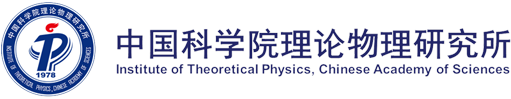(Lunch Seminar) Computation with Tensor Networks

CAS Key Laboratory of Theoretical Physics

Institute of Theoretical Physics

Lunch Seminar

Title

Computation with Tensor Networks

Speaker

 Pan Zhang

Affiliation

Date

4月20日12:00

Venue

6620

Contact Person

Abstract

In the lunch seminar, Pan Zhang will present methods and algorithms for solving statistical mechanics problems, combinatorial optimization problems, and quantum circuit simulations, in an integrated framework based on tensor networks.
In statistical mechanics problems, the partition function (i.e. the normalization factor of the Boltzmann distribution) at a finite temperature can be obtained by contracting a tensor network that is converted from the statistical mechanics problem. When equipped with the “Tropical” algebra, the tensor network contraction can be used to obtain ground state energy and entropy of the model directly at zero temperature. When the interactions in the statistical mechanics model are complex, computing the partition function acts as estimating the amplitude of an end state of a quantum circuit, thus tensor network contractions can be used to simulate quantum computers. Pan Zhang will introduce approximate and exact algorithms for contracting tensor networks, and their wide applications, particularly in simulating Google’s Sycamore quantum circuits.